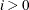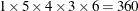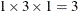The HPNLMOD Procedure

PARAMETERS Statement

Subsections:

• PARAMETERS <parameter-specification> <,…, parameter-specification> </ options>;

• PARMS <parameter-specification> <,…, parameter-specification> </ options>;

The purpose of the PARAMETERS statement is to provide starting values for the HPNLMOD procedure. You can provide values that define a single point in the parameter space or that define a set of points. For more information about parameter-specification, see the section Assigning Starting Values by Using Parameter Specification.

You can specify the following options in the PARAMETERS statement after the slash (/).

BEST=specifies the maximum number of parameter grid points and the corresponding objective function values to display in the “Parameters” table. If you specify this option, the parameter grid points are listed in ascending order of objective function value. By default, all parameter grid points are displayed.

PDATA=SAS-data-set
DATA=SAS-data-set

specifies the data set that provides parameter starting values.

START=value
DEFSTART=value

specifies a default starting value for all parameters.

There are four methods available for providing starting values to the optimization process. In descending order of precedence, the methods are as follows:

1. Specify values directly in the PARAMETERS statement.

2. Specify values in the PDATA= data set option.

3. Specify a single value for all parameters by using the START= option.

4. Use the default value 1.0.

The names that are assigned to parameters must be valid SAS names and must not coincide with names of variables in the input data set (see the DATA= option in the PROC HPNLMOD statement). Parameters that are assigned starting values through the PARAMETERS statement can be omitted from the estimation if the expression in the MODEL statement does not depend on them.

Assigning Starting Values by Using Parameter Specification

A parameter-specification has the following general form, where name identifies the parameter and value-list provides the set of starting values for the parameter:

• name = value-list

Often the value-list contains only a single value, but more general and flexible list specifications such as the following are possible:

m

a single value

m1, m2, …, mn

several values

m TO n

a sequence in which m equals the starting value, n equals the ending value, and the increment equals 1

m TO n BY i

a sequence in which m equals the starting value, n equals the ending value, and the increment is i

m1, m2 TO m3

mixed values and sequences

When you specify more than one value for a parameter, PROC HPNLMOD sorts the values in ascending sequence and removes duplicate values from the parameter list before forming the grid for the parameter search. If you specify several values for each parameter, PROC HPNLMOD evaluates the model at each point on the grid. The iterations then commence from the point on the grid that yields the smallest objective function value.

For example, the following PARAMETERS statement specifies five parameters and sets their possible starting values as shown in the following table:

parms  b0 = 0
b1 = 4 to 8
b2 = 0 to .6 by .2
b3 = 1, 10, 100
b4 = 0, .5, 1 to 4;


Table 10.2: continued

Possible Starting Values

B0

B1

B2

B3

B4

0

4

0.0

1

0.0

5

0.2

10

0.5

6

0.4

100

1.0

7

0.6

2.0

8

3.0

4.0

The objective function values are calculated for each of thecombinations of possible starting values. Each grid point’s objective function value is computed by using the execution mode that is specified in the PERFORMANCE statement.

If you specify a starting value by using a parameter-specification, any starting values that are provided for this parameter through the PDATA= data set are ignored. The parameter-specification overrides the information in the PDATA= data set.

Assigning Starting Values from a SAS Data Set

The PDATA= option in the PARAMETERS statement enables you to assign starting values for parameters by using a SAS data set. The data set must contain at least two variables: a character variable named Parameter or Parm that identifies the parameter, and a numeric variable named Estimate or Est that contains the starting values. For example, the PDATA= option enables you to use the contents of the "ParameterEstimates" table from one PROC HPNLMOD run to supply starting values for a subsequent run, as follows:

proc hpnlmod data=d(obs=30);
parameters alpha=100 beta=3 gamma=4;
Switch = 1/(1+gamma*exp(beta*log(dose)));
model y ~ residual(alpha*Switch);
ods output ParameterEstimates=pest;
run;

proc hpnlmod data=d;
parameters / pdata=pest;
Switch = 1/(1+gamma*exp(beta*log(dose)));
model y ~ residual(alpha*Switch);
run;


You can specify multiple values for a parameter in the PDATA= data set, and the parameters can appear in any order. The starting values are collected by parameter and arranged in ascending order, and duplicate values are removed. The parameter names in the PDATA= data set are not case sensitive. For example, the following DATA step defines starting values for three parameters and a starting grid withpoints:

data test;
input Parameter \$ Estimate;
datalines;
alpha  100
BETA   4
beta   4.1
beta    4.2
beta    4.1
gamma 30
;